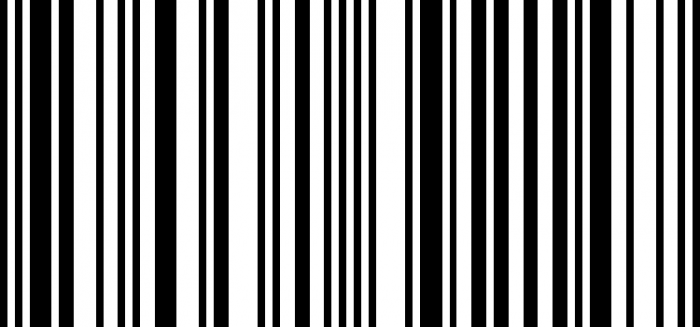# How to produce a barcode in Matplotlib?

MatplotlibPythonData Visualization

To produce a barcode in Matplotlib, we can take the following steps −

• Set the figure size and adjust the padding between and around the subplots.
• Make a list of binary numbers, i.e., 0s and 1s.
• Create a new figure or activate an existing figure with dpi=100
• Add an axes to the figure.
• Turn off the axes.
• Use imshow() method to plot the data from step 2.
• To display the figure, use show() method.

## Example

import matplotlib.pyplot as plt
import numpy as np

plt.rcParams["figure.figsize"] = [7.50, 3.50]
plt.rcParams["figure.autolayout"] = True

code = np.array([
1, 0, 1, 0, 1, 1, 1, 0, 1, 1, 0, 0, 0, 1, 0, 0, 1, 0, 1, 0, 0, 1, 1, 1,
0, 0, 0, 1, 0, 1, 1, 0, 0, 0, 0, 1, 0, 1, 0, 0, 1, 1, 0, 0, 1, 0, 1, 0,
1, 0, 1, 0, 0, 0, 0, 1, 0, 1, 1, 1, 0, 1, 0, 0, 1, 1, 0, 1, 1, 0, 0, 1,
1, 0, 0, 1, 1, 0, 1, 0, 1, 1, 1, 0, 0, 1, 0, 0, 0, 1, 0, 0, 1, 0, 1])

fig = plt.figure(dpi=100)

ax = fig.add_axes([0, 0, 1, 1])
ax.set_axis_off()

ax.imshow(code.reshape(1, -1), cmap='binary', aspect='auto',
interpolation='nearest')

plt.show()

## Output